# 1. A process is known to be first order in the response of the tank temperature,...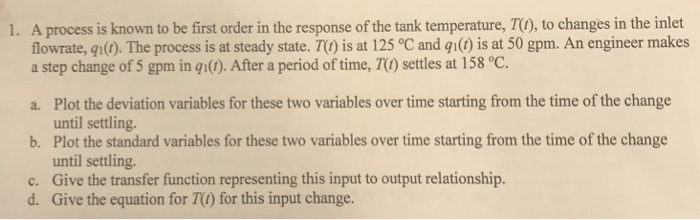1. A process is known to be first order in the response of the tank temperature, TO, to changes in the inlet flowrate, gi(). The process is at steady state. T) is at 125 °C and gi) is at 50 gpm. An engineer makes a step change of 5 gpm in qi(0. After a period of time, TO) settles at 158 °C Plot the deviation variables for these two variables over time starting from the time of the change until settling. Plot the standard variables for these two variables over time starting from the time of the change until settling. Give the transfer function representing this input to output relationship. Give the equation for TC) for this input change. a. b. c. d.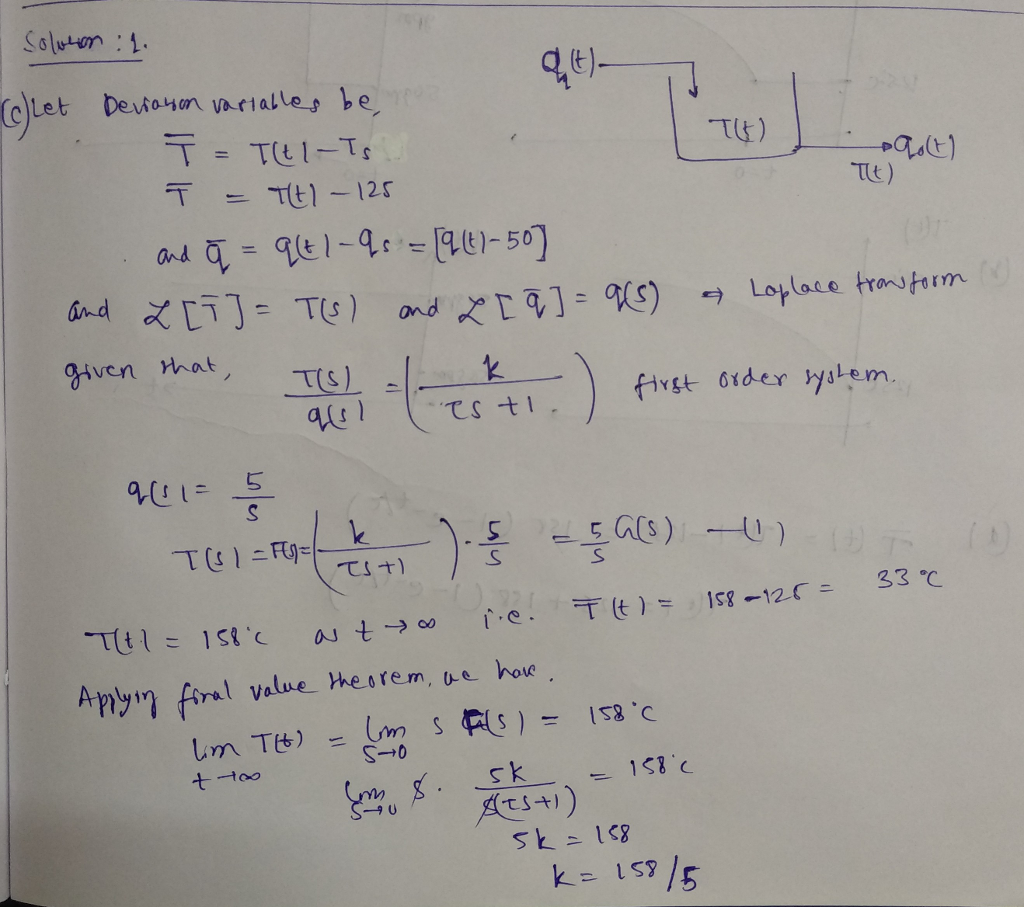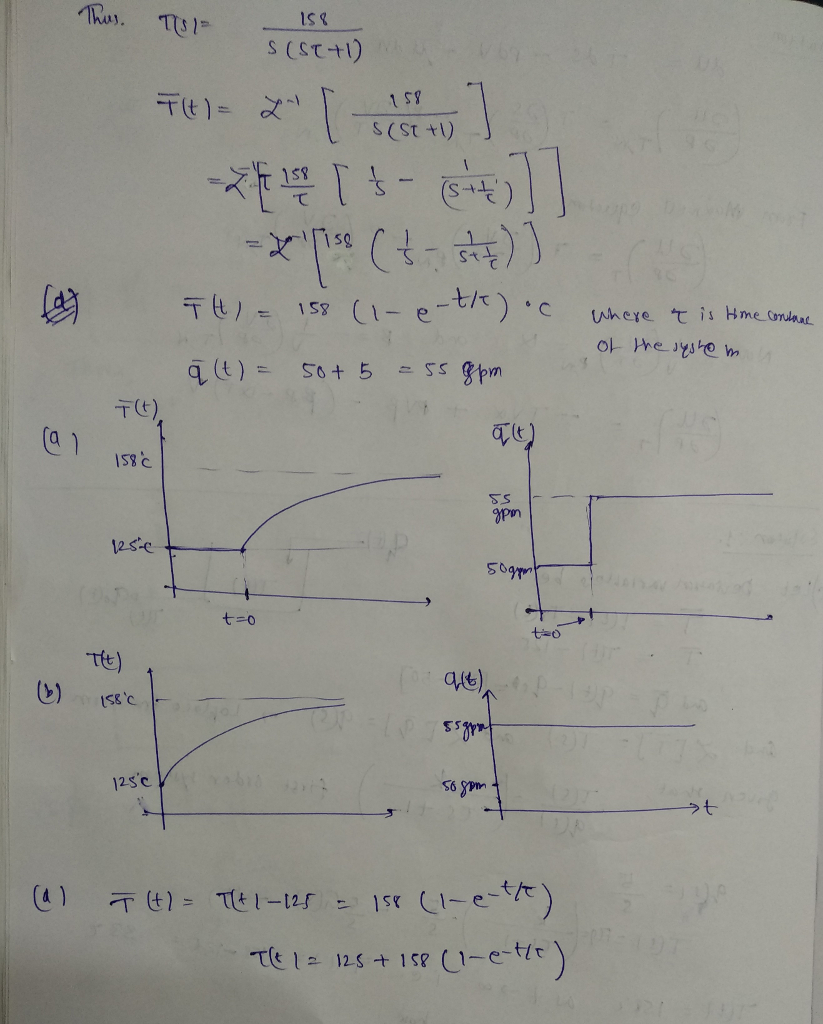#### Earn Coin

Coins can be redeemed for fabulous gifts.

Similar Homework Help Questions
• ### USING LAPLACE TRANSFORMS Question 1 Two non-interacting tanks are connected in series as shown in the...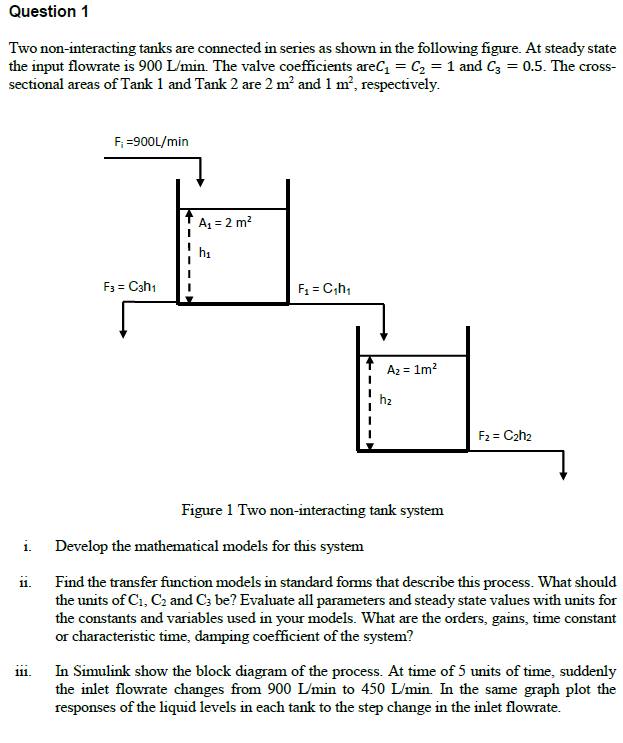USING LAPLACE TRANSFORMS Question 1 Two non-interacting tanks are connected in series as shown in the following figure. At steady state the input flow rate is 900 Limin. The valve coefficients areG = G = 1 and G = 0.5. The cross- sectional areas of Tank 1 and Tank 2 are 2 m2 and 1 m', respectively. Fi 900L/min hi h2 Figure 1 Two non-interacting tank system i. Develop the mathematical models for this system ii. Find the transfer function...

• ### Question 2 a) The following diagram show a liquid-level storage tank with q, and qi is...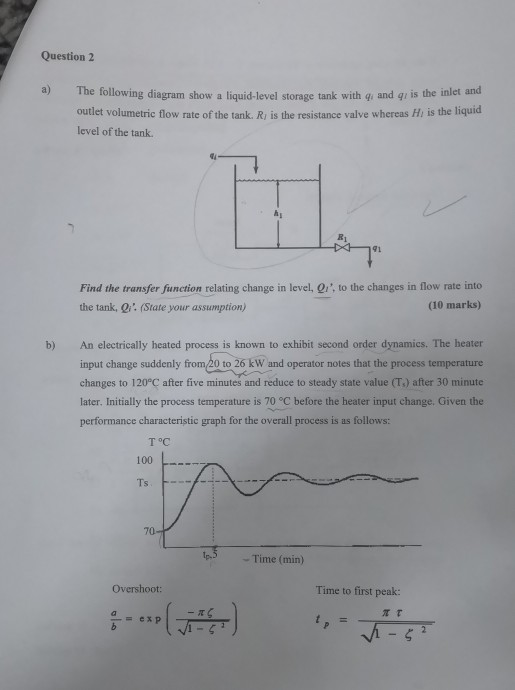Question 2 a) The following diagram show a liquid-level storage tank with q, and qi is the inlet and outlet volumetric flow rate of the tank. Ri is the resistance valve whereas Hi is the liquid level of the tank. Find the transfer function relating change in level, Qi', to the changes in flow rate into the tank, Q' (State your assumption) (10 marks) b) An electrically heated process is known to exhibit second order dynamics. The heater input change...

• ### The level ho of liquid in a vertical cylindrical tank as shown in Figure 4 is related to the infl...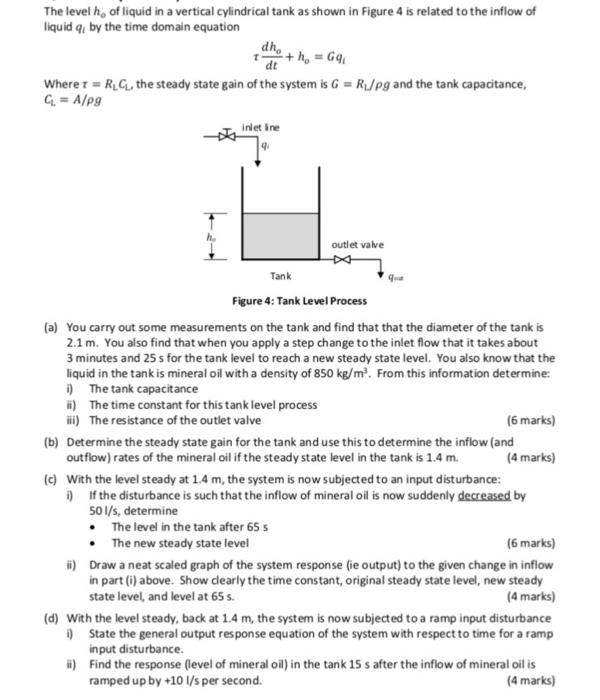please answer all of part i really need them ASAP ... The level ho of liquid in a vertical cylindrical tank as shown in Figure 4 is related to the inflow of liquid qi by the time domain equation d h dt where τ RLGL, the steady state gain of the system is G RL/pg and the tank capacitance, inlet ine ho outlet vave Tank Figure 4: Tank Level Process (a) You carry out some measurements on the tank and...

• ### Question A1 A single tank process has first order dynamics. When the inlet flow rate is...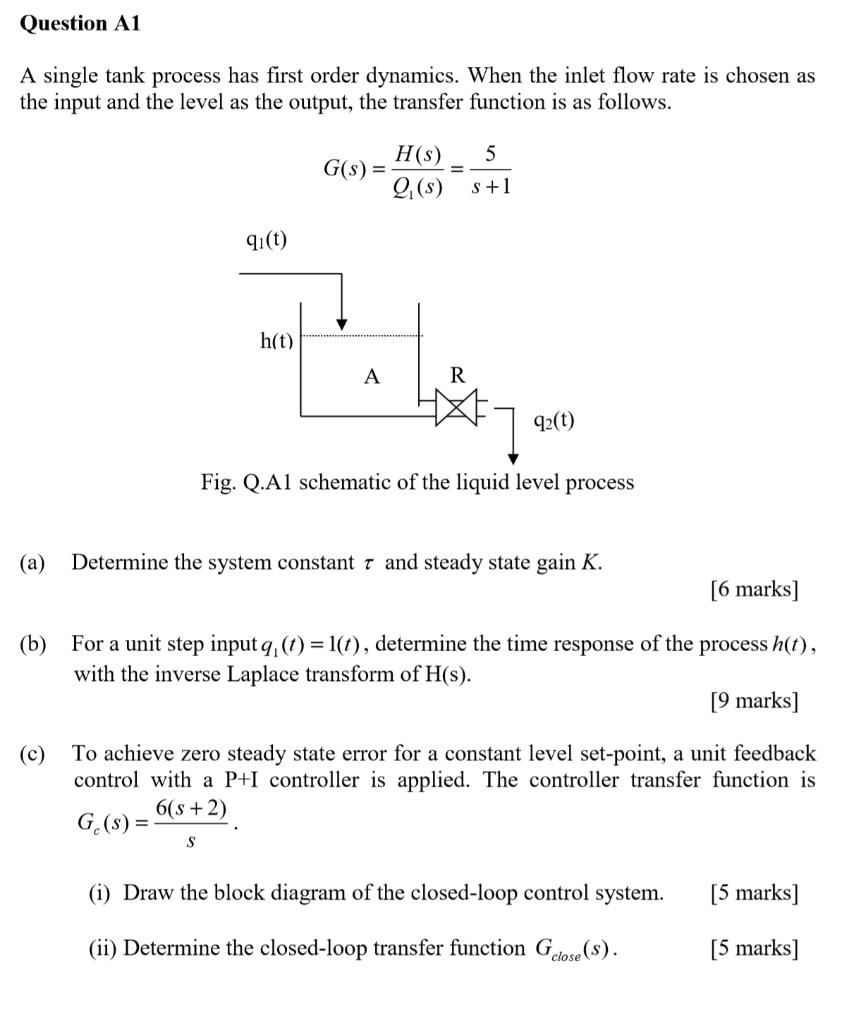Question A1 A single tank process has first order dynamics. When the inlet flow rate is chosen as the input and the level as the output, the transfer function is as follows. 5 G(s) - H(s) 2. (S) S +1 91(t) h(t) *7 q:(0) q2(t) Fig. Q.Al schematic of the liquid level process (a) Determine the system constant t and steady state gain K. [6 marks] (b) For a unit step input q, (t) = 1(1), determine the time response...

• ### As a process engineer with the Complex Pole Corporation, you are assigned a unit with an exothermic chemical reacto...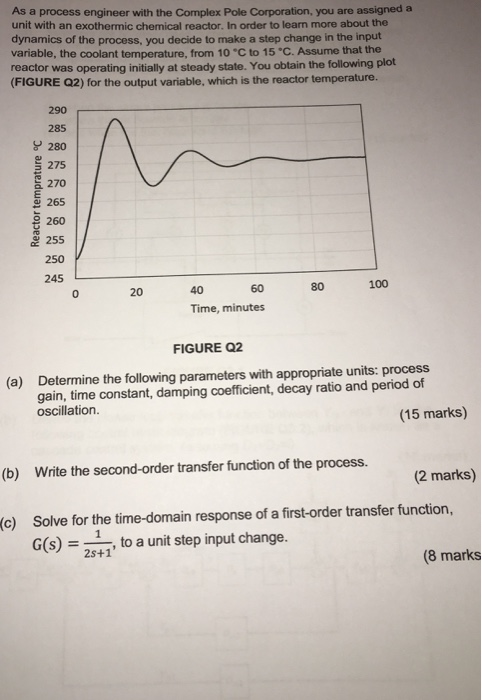As a process engineer with the Complex Pole Corporation, you are assigned a unit with an exothermic chemical reactor, In order to learn more about the dynamics of the process, you decide to make a step change in the input variable, the coolant temperature, from 10 °C to 15 C. Assume that the reactor was operating initially at steady state. You obtain the following plot (FIGURE Q2) for the output variable, which is the reactor temperature. 290 285 280 275...

• ### Problems 1. The following equation describes the process of warming a hot tub by adding hot...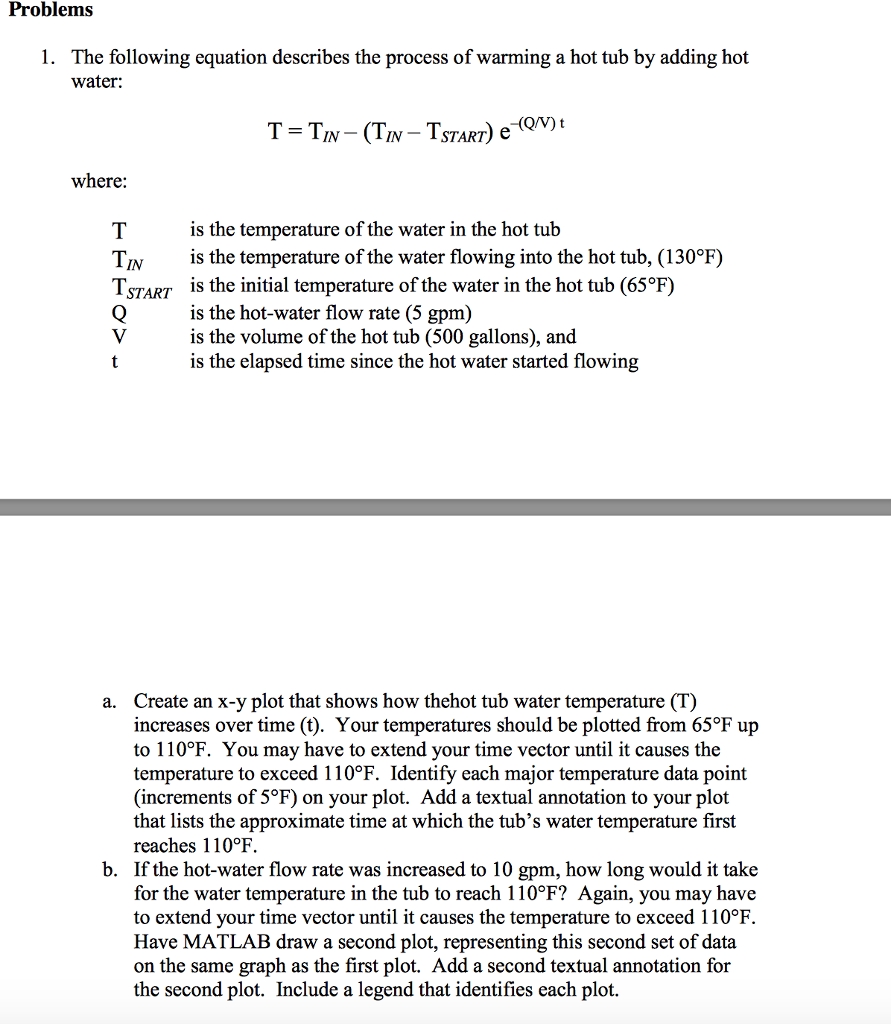Problems 1. The following equation describes the process of warming a hot tub by adding hot water: IN-ISTART) where is the temperature of the water in the hot tub is the temperature of the water flowing into the hot tub, (130°F) is the initial temperature of the water in the hot tub (65°F) is the hot-water flow rate (5 gpm) is the volume of the hot tub (500 gallons), and is the elapsed time since the hot water started flowing...

• ### (1) Steady state is when a. All process variables Temperature (T),Pressure(P), volumetric flowrate and mass do...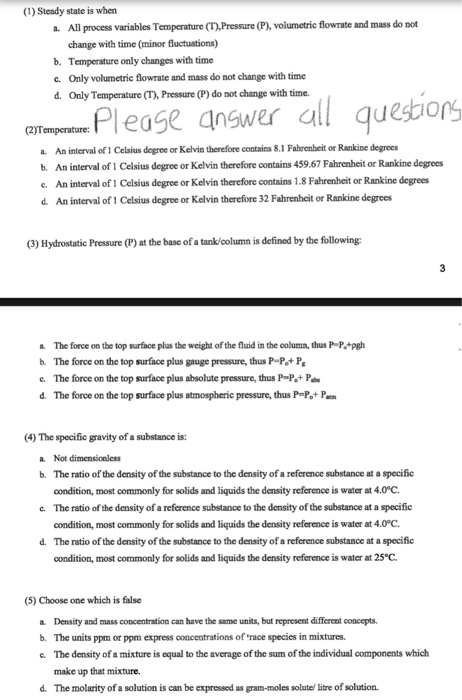(1) Steady state is when a. All process variables Temperature (T),Pressure(P), volumetric flowrate and mass do not change with time (minor fluctuations) b. Temperature only changes with time c. Only volumetric flowrate and mass do not change with time d. Only Temperature (T), Pressure (P) do not change with time. (2)Temperature: a. b. e. d. An interval of1 Celsius degree or Kelvin therefore contains 8.1 Fahrenbeit or Rankine degrees An interval of 1 Celsius degree or Kelvin therefore contains 459.67...

• ### Section 7.1 Introduction First Order Systems: Problem 5 Previous Problem Probiem List Next Problem (1 poing) Consider two interconnected tanks as shown in the figure above. Tank 1 Initial contain...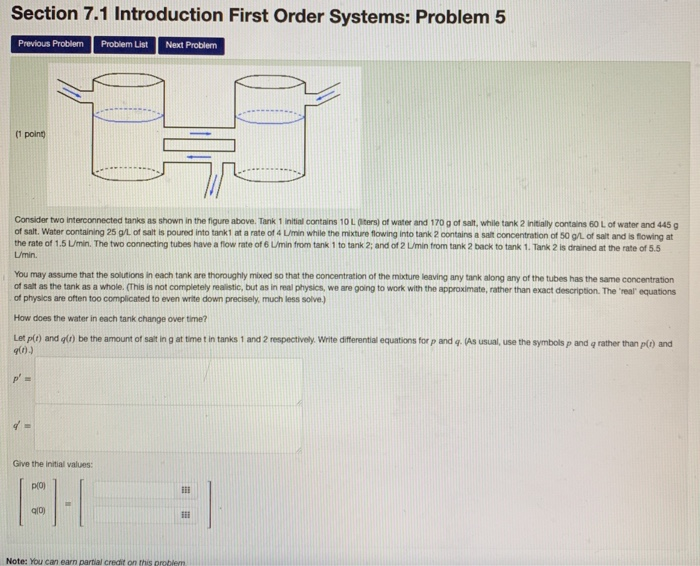Section 7.1 Introduction First Order Systems: Problem 5 Previous Problem Probiem List Next Problem (1 poing) Consider two interconnected tanks as shown in the figure above. Tank 1 Initial contains 10 L Olters) of water and 170 g of salt. while tank 2 Initiaily contains 60 L of water and 445 g of salt. Water containing 25 g'L of salt is poured into tank1 at a rate of 4 U/min while the mixture flowing into tank 2 contains a salt...

• ### Based on the data of Respirstion of Peas at Room Temperature and Colder Temperature answer the...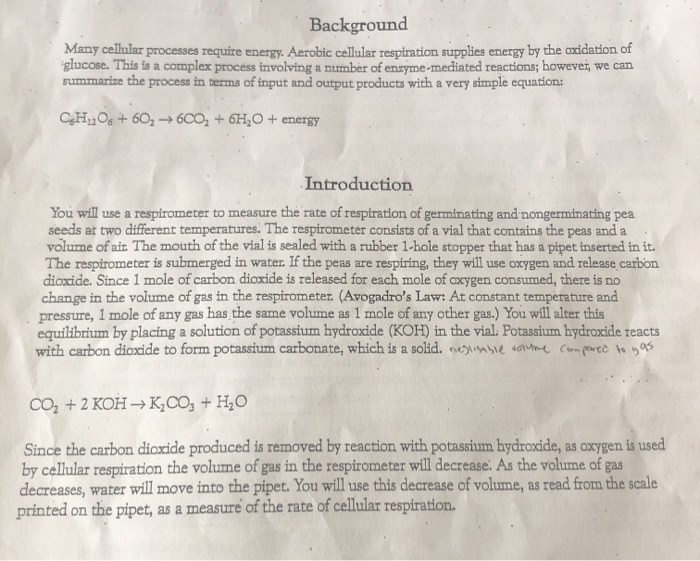Based on the data of Respirstion of Peas at Room Temperature and Colder Temperature answer the following questions: 1. Write two hypothesis that this experiment is designed to test 2. Using the general gas law (V= nRT/p) give the variables that had to be controlled for your data to be valid. State the controls used for each variable and any means used to correct for the influence of a variable. 3. Why does respirometer 3 serve as a negative control?...

• ### Implement a tic-tac-toe game. At the bottom of these specifications you will see a template you...

Implement a tic-tac-toe game. At the bottom of these specifications you will see a template you must copy and paste to cloud9. Do not change the provided complete functions, or the function stub headers / return values. Currently, if the variables provided in main are commented out, the program will compile. Complete the specifications for each function. As you develop the program, implement one function at a time, and test that function. The provided comments provide hints as to what...